# Algebric Functions.pdf

2 de Jun de 2023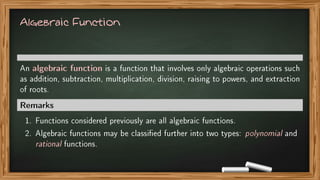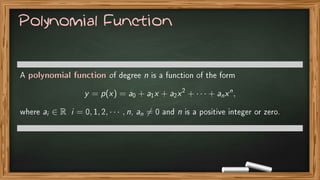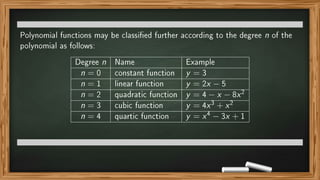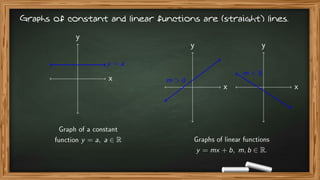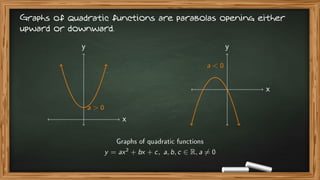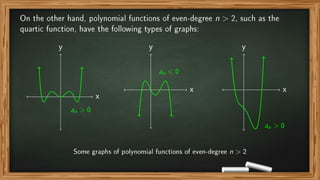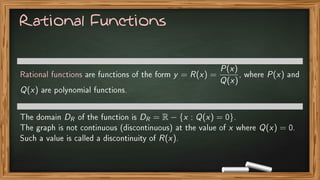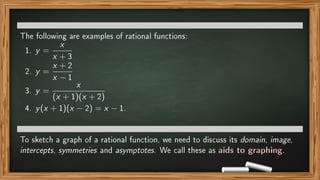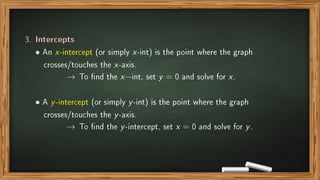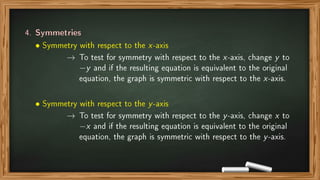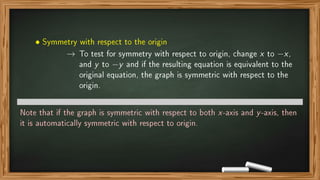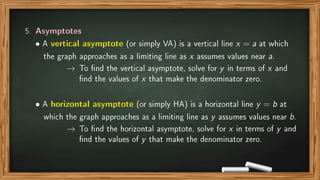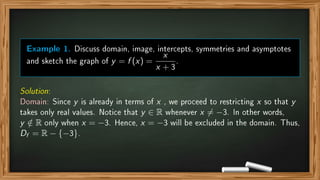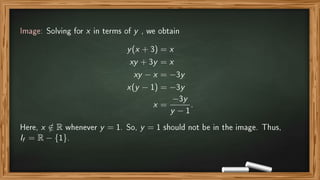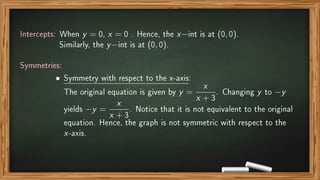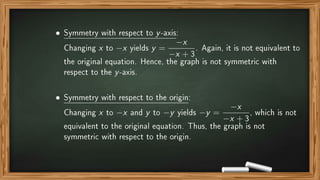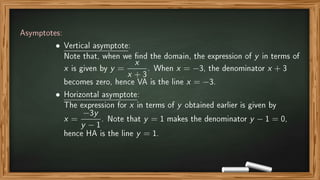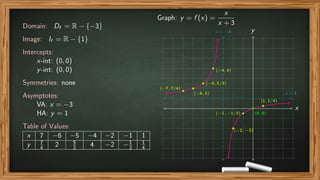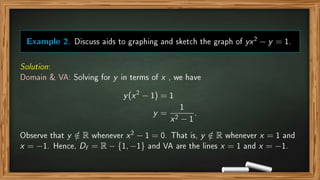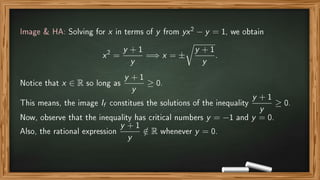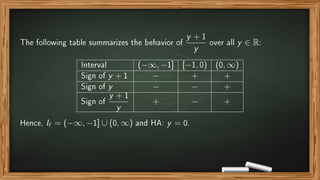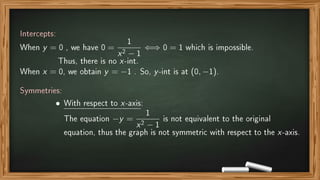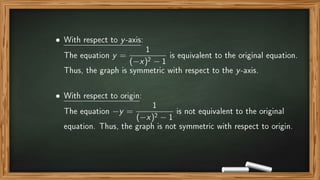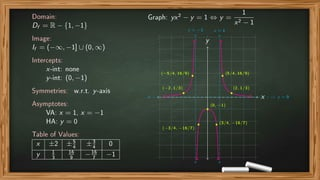1 de 28

### Algebric Functions.pdf

• 1. ALGEBRAIC FUNCTIONS (MAT049 - PreCalculus) 1st Semester, 2021-2022 Maribeth B. Montero, PhD Mathematics Department Mindanao State University Main Campus Marawi City maribeth.montero@msumain.edu.ph
• 2. Algebraic Function An algebraic function is a function that involves only algebraic operations such as addition, subtraction, multiplication, division, raising to powers, and extraction of roots. Remarks 1. Functions considered previously are all algebraic functions. 2. Algebraic functions may be classied further into two types: polynomial and rational functions.
• 3. Polynomial Function A polynomial function of degree n is a function of the form y = p(x) = a0 + a1x + a2x2 + · · · + anxn , where ai ∈ R i = 0, 1, 2, · · · , n, an 6= 0 and n is a positive integer or zero.
• 4. Polynomial functions may be classied further according to the degree n of the polynomial as follows: Degree n Name Example n = 0 constant function y = 3 n = 1 linear function y = 2x − 5 n = 2 quadratic function y = 4 − x − 8x2 n = 3 cubic function y = 4x3 + x2 n = 4 quartic function y = x4 − 3x + 1
• 5. Graphs of constant and linear functions are (straight) lines. x y y = a Graph of a constant function y = a, a ∈ R x y m 0 x y m 0 Graphs of linear functions y = mx + b, m, b ∈ R.
• 6. Graphs of quadratic functions are parabolas opening either upward or downward. x y a 0 x y a 0 Graphs of quadratic functions y = ax2 + bx + c, a, b, c ∈ R, a 6= 0
• 7. In general, polynomial functions of odd-degree n 1, such as the cubic polynomials, have the following types of graphs. x y an 0 x y an 0 x y an 0 Some graphs of polynomial functions of odd-degree n 1
• 8. On the other hand, polynomial functions of even-degree n 2, such as the quartic function, have the following types of graphs: x y an 0 x y an 0 x y an 0 Some graphs of polynomial functions of even-degree n 2
• 9. Rational Functions Rational functions are functions of the form y = R(x) = P(x) Q(x) , where P(x) and Q(x) are polynomial functions. The domain DR of the function is DR = R − {x : Q(x) = 0}. The graph is not continuous (discontinuous) at the value of x where Q(x) = 0. Such a value is called a discontinuity of R(x).
• 10. The following are examples of rational functions: 1. y = x x + 3 2. y = x + 2 x − 1 3. y = x (x + 1)(x + 2) 4. y(x + 1)(x − 2) = x − 1. To sketch a graph of a rational function, we need to discuss its domain, image, intercepts, symmetries and asymptotes. We call these as aids to graphing.
• 11. Aids to Graphing 1. Domain → To nd the domain, solve for y in terms of x and restrict x so that y takes only real values. 2. Image → To nd the image, solve for x in terms of y and restrict y so that x takes only real values.
• 12. 3. Intercepts • An x-intercept (or simply x-int) is the point where the graph crosses/touches the x-axis. → To nd the x−int, set y = 0 and solve for x. • A y-intercept (or simply y-int) is the point where the graph crosses/touches the y-axis. → To nd the y-intercept, set x = 0 and solve for y.
• 13. 4. Symmetries • Symmetry with respect to the x-axis → To test for symmetry with respect to the x-axis, change y to −y and if the resulting equation is equivalent to the original equation, the graph is symmetric with respect to the x-axis. • Symmetry with respect to the y-axis → To test for symmetry with respect to the y-axis, change x to −x and if the resulting equation is equivalent to the original equation, the graph is symmetric with respect to the y-axis.
• 14. • Symmetry with respect to the origin → To test for symmetry with respect to origin, change x to −x, and y to −y and if the resulting equation is equivalent to the original equation, the graph is symmetric with respect to the origin. Note that if the graph is symmetric with respect to both x-axis and y-axis, then it is automatically symmetric with respect to origin.
• 15. 5. Asymptotes • A vertical asymptote (or simply VA) is a vertical line x = a at which the graph approaches as a limiting line as x assumes values near a. → To nd the vertical asymptote, solve for y in terms of x and nd the values of x that make the denominator zero. • A horizontal asymptote (or simply HA) is a horizontal line y = b at which the graph approaches as a limiting line as y assumes values near b. → To nd the horizontal asymptote, solve for x in terms of y and nd the values of y that make the denominator zero.
• 16. Example 1. Discuss domain, image, intercepts, symmetries and asymptotes and sketch the graph of y = f (x) = x x + 3 . Solution: Domain: Since y is already in terms of x , we proceed to restricting x so that y takes only real values. Notice that y ∈ R whenever x 6= −3. In other words, y / ∈ R only when x = −3. Hence, x = −3 will be excluded in the domain. Thus, Df = R − {−3}.
• 17. Image: Solving for x in terms of y , we obtain y(x + 3) = x xy + 3y = x xy − x = −3y x(y − 1) = −3y x = −3y y − 1 . Here, x / ∈ R whenever y = 1. So, y = 1 should not be in the image. Thus, If = R − {1}.
• 18. Intercepts: When y = 0, x = 0 . Hence, the x−int is at (0, 0). Similarly, the y−int is at (0, 0). Symmetries: • Symmetry with respect to the x-axis: The original equation is given by y = x x + 3 . Changing y to −y yields −y = x x + 3 . Notice that it is not equivalent to the original equation. Hence, the graph is not symmetric with respect to the x-axis.
• 19. • Symmetry with respect to y-axis: Changing x to −x yields y = −x −x + 3 . Again, it is not equivalent to the original equation. Hence, the graph is not symmetric with respect to the y-axis. • Symmetry with respect to the origin: Changing x to −x and y to −y yields −y = −x −x + 3 , which is not equivalent to the original equation. Thus, the graph is not symmetric with respect to the origin.
• 20. Asymptotes: • Vertical asymptote: Note that, when we nd the domain, the expression of y in terms of x is given by y = x x + 3 . When x = −3, the denominator x + 3 becomes zero, hence VA is the line x = −3. • Horizontal asymptote: The expression for x in terms of y obtained earlier is given by x = −3y y − 1 . Note that y = 1 makes the denominator y − 1 = 0, hence HA is the line y = 1.
• 21. Domain: Df = R − {−3} Image: If = R − {1} Intercepts: x-int: (0, 0) y-int: (0, 0) Symmetries: none Asymptotes: VA: x = −3 HA: y = 1 Table of Values: x 7 −6 −5 −4 −2 −1 1 y 7 4 2 5 2 4 −2 −1 2 1 4 Graph: y = f (x) = x x + 3 x y x = −3 y = 1 • (−7, 7/4) • (−6, 2) • (−5, 5/2) • (−4, 4) •(−2, −2) • (−1, −1/2) • (0, 0) • (1, 1/4)
• 22. Example 2. Discuss aids to graphing and sketch the graph of yx2 − y = 1. Solution: Domain VA: Solving for y in terms of x , we have y(x2 − 1) = 1 y = 1 x2 − 1 . Observe that y / ∈ R whenever x2 − 1 = 0. That is, y / ∈ R whenever x = 1 and x = −1. Hence, Df = R − {1, −1} and VA are the lines x = 1 and x = −1.
• 23. Image HA: Solving for x in terms of y from yx2 − y = 1, we obtain x2 = y + 1 y =⇒ x = ± r y + 1 y . Notice that x ∈ R so long as y + 1 y ≥ 0. This means, the image If constitues the solutions of the inequality y + 1 y ≥ 0. Now, observe that the inequality has critical numbers y = −1 and y = 0. Also, the rational expression y + 1 y / ∈ R whenever y = 0.
• 24. The following table summarizes the behavior of y + 1 y over all y ∈ R: Interval (−∞, −1] [−1, 0) (0, ∞) Sign of y + 1 − + + Sign of y − − + Sign of y + 1 y + − + Hence, If = (−∞, −1] ∪ (0, ∞) and HA: y = 0.
• 25. Intercepts: When y = 0 , we have 0 = 1 x2 − 1 ⇐⇒ 0 = 1 which is impossible. Thus, there is no x-int. When x = 0, we obtain y = −1 . So, y-int is at (0, −1). Symmetries: • With respect to x-axis: The equation −y = 1 x2 − 1 is not equivalent to the original equation, thus the graph is not symmetric with respect to the x-axis.
• 26. • With respect to y-axis: The equation y = 1 (−x)2 − 1 is equivalent to the original equation. Thus, the graph is symmetric with respect to the y-axis. • With respect to origin: The equation −y = 1 (−x)2 − 1 is not equivalent to the original equation. Thus, the graph is not symmetric with respect to origin.
• 27. Domain: Df = R − {1, −1} Image: If = (−∞, −1] ∪ (0, ∞) Intercepts: x-int: none y-int: (0, −1) Symmetries: w.r.t. y-axis Asymptotes: VA: x = 1, x = −1 HA: y = 0 Table of Values: x ±2 ±5 4 ±3 4 0 y 1 3 16 9 −16 7 −1 Graph: yx2 − y = 1 ⇔ y = 1 x2 − 1 x y x = −1 x = 1 y = 0 • (0, −1) • (2, 1/3) • (−2, 1/3) • (5/4, 16/9) • (−5/4, 16/9) • (3/4, −16/7) • (−3/4, −16/7)
• 28. Exercises Discuss the aids to graphing and sketch the graph of each of the following. 1. y = −2 x + 1 2. y = 2 x2 − x 3. y = x (x − 1)(x + 2) 4. y(x + 1)(x − 2) = x − 1.